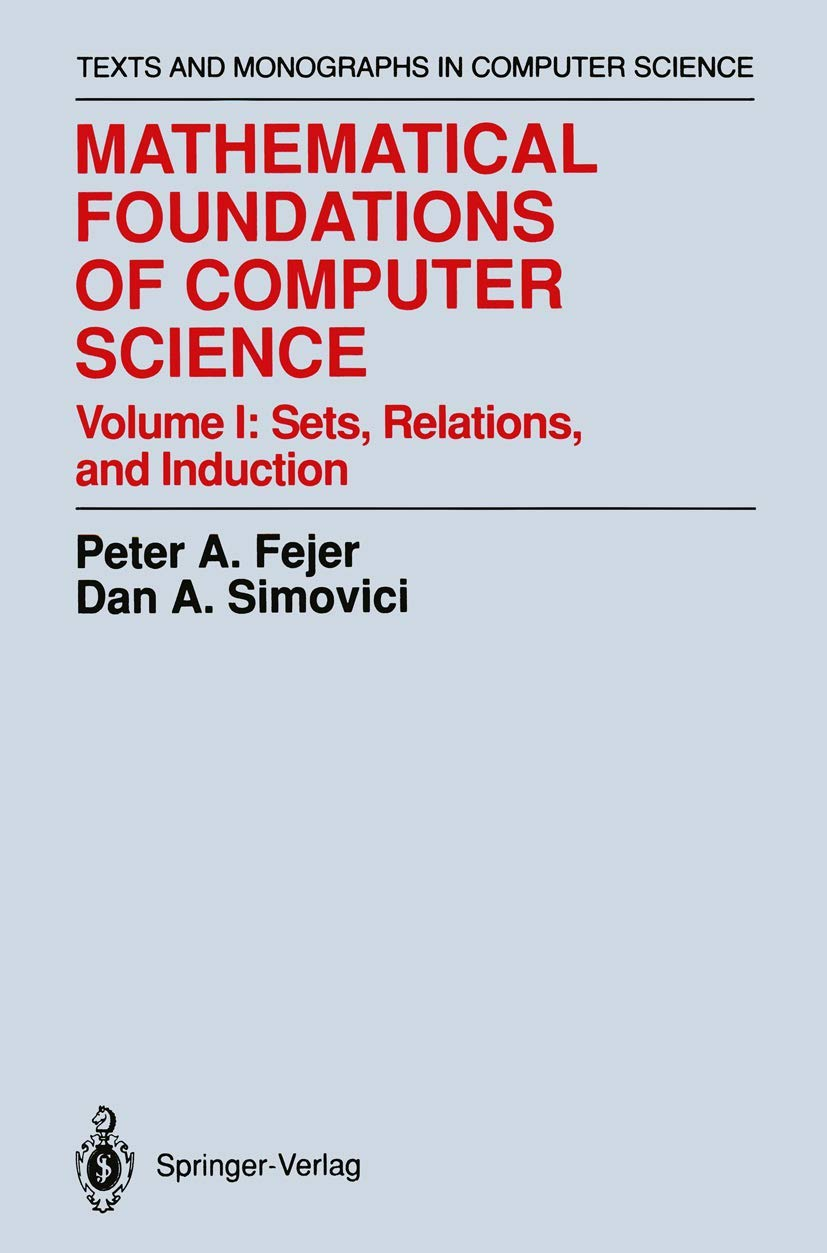# Mathematical Foundations of Computer Science: Sets, Relations, and Induction: v. 1 (Monographs in Computer Science)Price: ₹2,355.10
(as of Oct 23,2021 18:27:19 UTC – Details)Mathematical Foundations of Computer Science, Volume I is the first of two volumes presenting topics from mathematics (mostly discrete mathematics) which have proven relevant and useful to computer science. This volume treats basic topics, mostly of a set-theoretical nature (sets, functions and relations, partially ordered sets, induction, enumerability, and diagonalization) and illustrates the usefulness of mathematical ideas by presenting applications to computer science. Readers will find useful applications in algorithms, databases, semantics of programming languages, formal languages, theory of computation, and program verification. The material is treated in a straightforward, systematic, and rigorous manner. The volume is organized by mathematical area, making the material easily accessible to the upper-undergraduate students in mathematics as well as in computer science and each chapter contains a large number of exercises. The volume can be used as a textbook, but it will also be useful to researchers and professionals who want a thorough presentation of the mathematical tools they need in a single source. In addition, the book can be used effectively as supplementary reading material in computer science courses, particularly those courses which involve the semantics of programming languages, formal languages and automata, and logic programming.

Scroll to Top